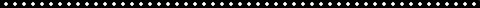SCHOLARLY INTERESTSI am primarily interested in numerical analysis and applied mathematics. The title of the volume my first published paper appears in says it best: Math Is For Solving Problems (Editors L. Pamela Cook and Victor Roytburd, SIAM, 1996).

My thesis work (under Julian D. Cole and Don W. Schwendeman) was in theoretical aerodynamics/ scientific computing. There I showed that it was possible to compute solutions of the Transonic Small Disturbance equations that in the case of flow around a slender body of revolution without fore-aft symmetry possessed vanishingly small shocks. This was a very tricky calculation because it involves trying to obtain the solution accurately as near as one can get to a rather nasty singularity. In 1998, I was invited to present these results at the 2nd Theoretical Fluid Mechanics meeting at the annual American Institute of Aeronautics and Astronautics conference. Along the way we stumbled upon an interesting way to discretize the radial derivatives in our partial differential equation. I later discovered that Professor Ron Mickens of Clark Atlanta University had been writing about these same kinds of "nonstandard finite difference" schemes for years.

I have always been interested in applying mathematics to unusual phenomena so when I had a sabbatical coming up in Spring 2000 I suggested to my colleague David Edwards that we come up with a new mathematical model for "how movies make money." I call this area "cinematic box-office dynamics" and have written two papers on the topic (Edwards and Buckmire, 2001; Edwards, Buckmire and Ortega-Gingrich, 2013) and conducted a lot of research (which sadly has not resulted in publication, yet).

Most of my mathematical work has been investigating nonstandard finite difference schemes, particularly Mickens discretizations of the radial operators in the cylindrical and spherical Laplacian operators.

A famous old problem, called the Bratu problem, is a nonlinear version of Helmholtz equation where the right hand side of
Laplacian u = - f is exponential. In cylindrical and planar coordinates there are known exact solutions to the Bratu problem, making it ideal for numerical benchmarking of my nonstandard finite difference scheme(s).

More recently I have expanded my work to venture into the area of data science. Specifically, applying macbine learning teachniques to produce mathematical models of the  commitment decisions of students who are admitted to colleges.

DATA SCIENCE

NONSTANDARD FINITE DIFFERENCES

MATHEMATICAL MODELLING

CLASSROOM VOTING / PEER INSTRUCTION

DIVERSITY, EQUITY & INCLUSION IN MATHEMATICS

COMPUTATIONAL AERODYNAMICS

GENDER, SEXUALITY and THE LAW

VISUALIZATION OF CONCEPT MAPS

• October 2001: I have become interested in doing mathematical education research on how students visualize Concept Maps in Linear Algebra. Here are some of the Concept Maps that I have produced so far.

UNDERREPRESENTATION IN MATHEMATICS

• 2005-present: I have been involved in a long-term project to improve mathematics (primarily algebra) instruction in northeast Los Angeles schools. I co-created and co-taught a  class Mathematics, Education and Access To Power multiple times to give students a community-based learning experience in mathematics.
• April 2010: "A Black, Gay mathematics department chair? Or, How I Got Here From There" (Friday April 2, 2010, Seminar on Underrepresentation in the Mathematical Sciences, Harvey Mudd College)Ron Buckmire